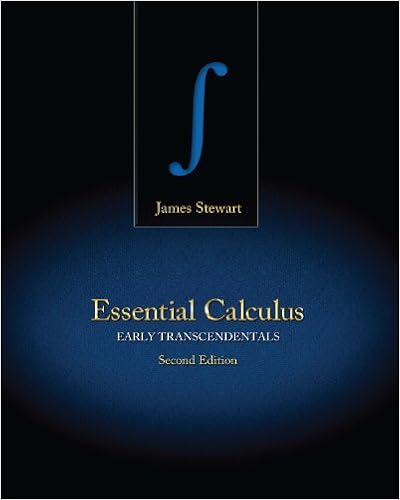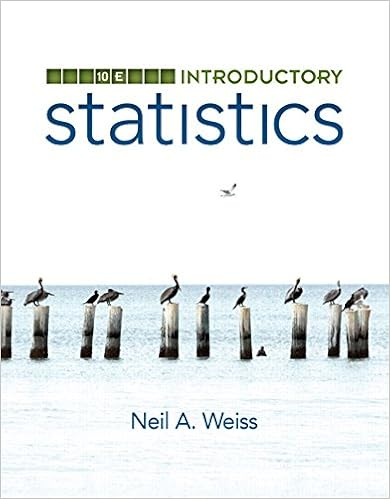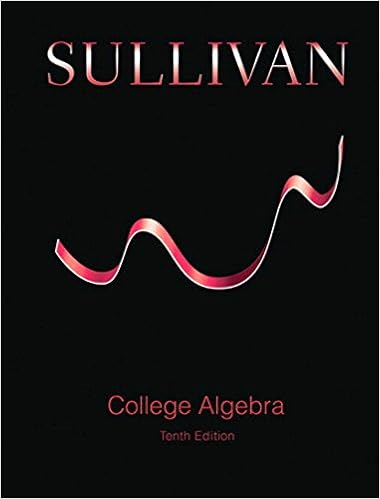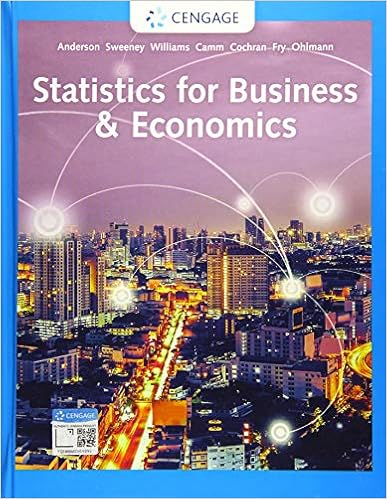# big ideas - algebra 2 - chapter 8.pdf - 8 8.1 8.2 8.3 8.4...

• Assessment
• 52

This preview shows page 1 - 4 out of 52 pages.

8.1Defining and Using Sequences and Series8.2Analyzing Arithmetic Sequences and Series8.3Analyzing Geometric Sequences and Series8.4Finding Sums of Infinite Geometric Series8.5Using Recursive Rules with Sequences8Sequences and SeriesMarching Band(p. 423)Skydiving(p. 431)Tree Farm(p. 449)Fish Population(p. 445)Museum Skylight(p. 416)MarchingBand(p.423)MuseumSkylight(p 416)()Tree Farm(p.449)Fi h Pl ti(445)SEE the Big IdeaChapter Learning Target:Understand sequences and series.Chapter Success Criteria:I can define and use sequences and series.I can describe how to find sums of infinitegeometric series.I can analyze arithmetic and geometricsequences and series.I can explain how to write recursive rulesfor sequences.
##### We have textbook solutions for you!The document you are viewing contains questions related to this textbook.
Chapter 8 / Exercise 4
Essential Calculus: Early Transcendentals
StewartExpert VerifiedBrowse all Textbook Solutions
407Maintaining Mathematical ProficiencyMaintaining Mathematical ProficiencyEvaluating FunctionsExample 1Evaluate the functiony=2x210 for the valuesx=0, 1, 2, 3, and 4.Input,x2x210Output,y02(0)2101012(1)210822(2)210232(3)210842(4)21022Copy and complete the table to evaluate the function.1.y=32x2.y=5x2+13.y=4x+24xy123xy234xy51015Solving EquationsExample 2Solve the equation 45=5(3)x.45=5(3)xWrite original equation.455=5(3)x5Divide each side by 5.9=3xSimplify.log39=log33xTake log3of each side.2=xSimplify.Solve the equation. Check your solution(s).4.7x+3=315.116=4(12)x6.216=3(x+6)7.2x+16=1448.14x8=179.8(34)x=27810.ABSTRACT REASONINGThe graph of the exponential decay functionf(x)=bxhas anasymptote y=0. How is the graph offdifferent from a scatter plot consisting of the points(1, b1), (2,b1+b2), (3,b1+b2+b3), . . .? How is the graph offsimilar?Dynamic Solutions available atBigIdeasMath.com

Course Hero member to access this document

Course Hero member to access this document

End of preview. Want to read all 52 pages?

Course Hero member to access this document

Term
Spring
Professor
Graham,L
Tags
Geometric progression, Mathh
##### We have textbook solutions for you!
The document you are viewing contains questions related to this textbook.The document you are viewing contains questions related to this textbook.
Chapter 8 / Exercise 4
Essential Calculus: Early Transcendentals
StewartExpert Verified
•••